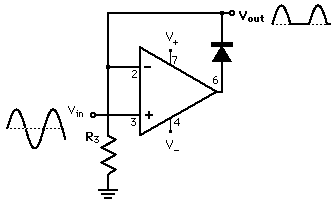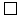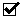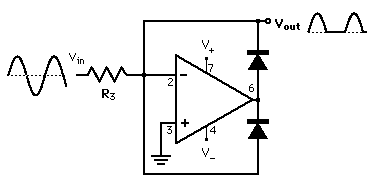# Precision Diode

Putting a diode in the feedback path produces a diode which gives virtually zero output for half a cycle and a gain of unity for the other half cycle. It can therefore rectify signals which are smaller than a diode drop. This device would usually be followed by a voltage follower.

The high frequency response of this device is limited by the slew rate of the op-amp used. Distortion can occur if the op-amp swings to its negative supply voltage and can't get back fast enough. In this case the output will stay at zero too long, distorting the wave.

 Rules application
 High-frequency modificationMenuPrecision diodeIntegratorDifferentiator
Index

Electronics concepts

Op-amp concepts

 HyperPhysics*****Electricity and magnetism R Nave
Go Back

# Rule Application: Precision Diode

 This can be considered to be a non-inverting amplifier with a diode in the feedback path. When the input goes negative, the output of the op-amp goes to the negative supply so that no current flows and the output is clamped to zero. When the signal goes positive the diode conducts to obey the current rule and the output tracks the input. Note that this is an example where the voltage rule is broken on the non-conducting half cycle. The gain is very high because of the reversed diode, but it can only drive the op-amp to the negative supply and cannot make the inputs equal in voltage.Index

Electronics concepts

Op-amp concepts

 HyperPhysics*****Electricity and magnetism R Nave
Go Back

# High Frequency Precision Diode

 This high frequency version of the precision diode relieves the slew rate limitations by preventing the op-amp from swinging to its negative supply voltage. This device would usually be followed by a voltage follower.Index

Electronics concepts

Op-amp concepts

 HyperPhysics*****Electricity and magnetism R Nave
Go Back